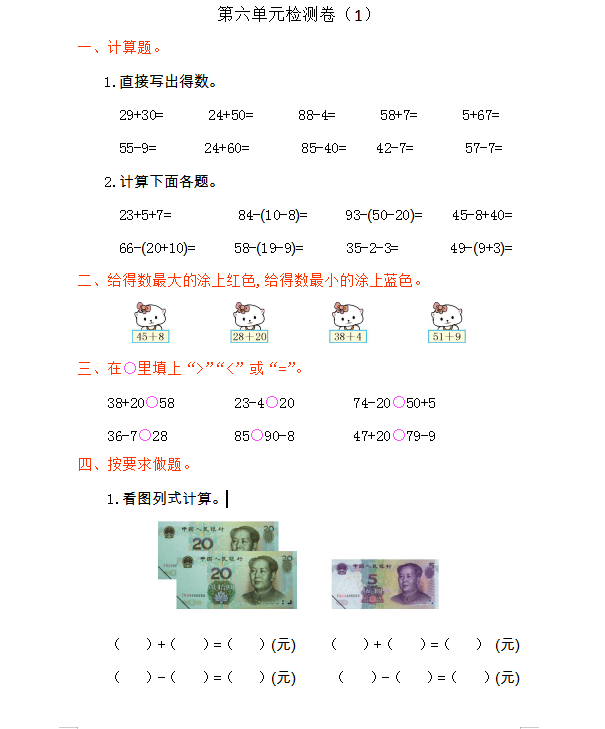1.直接写出得数。

29+30=　　　24+50=　　　88-4=　　　58+7=　　　5+67=

55-9=                24+60=           85-40=           42-7=                57-7=